# NCERT solutions for Mathematics Exemplar Class 11 chapter 6 - Linear Inequalities [Latest edition]

#### Chapters## Chapter 6: Linear Inequalities

Solved ExamplesExercise
Solved Examples [Pages 100 - 106]

### NCERT solutions for Mathematics Exemplar Class 11 Chapter 6 Linear Inequalities Solved Examples [Pages 100 - 106]

Solved Examples | Q 1.(i) | Page 100

Solve the inequality, 3x – 5 < x + 7, when x is a natural number

Solved Examples | Q 1.(ii) | Page 100

Solve the inequality, 3x – 5 < x + 7, when x is a whole number

Solved Examples | Q 1.(iii) | Page 100

Solve the inequality, 3x – 5 < x + 7, when x is an integer

Solved Examples | Q 1.(iv) | Page 100

Solve the inequality, 3x – 5 < x + 7, when x is a real number

Solved Examples | Q 2 | Page 100

Solve (x - 2)/(x + 5) > 2

Solved Examples | Q 3 | Page 101

Solve |3 – 4x| ≥ 9

Solved Examples | Q 4 | Page 101

Solve 1 ≤ |x –2| ≤ 3.

Solved Examples | Q 5 | Page 101

The cost and revenue functions of a product are given by C(x) = 20x + 4000 and R(x) = 60x + 2000, respectively, where x is the number of items produced and sold. How many items must be sold to realise some profit?

Solved Examples | Q 6 | Page 101

Solve for x, |x + 1| + |x| > 3.

Solved Examples | Q 7 | Page 102

Solve for x, (|x + 3| + x)/(x + 2) > 1

Solved Examples | Q 8 | Page 103

Solve the following system of inequalities:

x/(2x + 1) ≥ 1/4, (6x)/(4x - 1) < 1/2

Solved Examples | Q 9 | Page 104

Find the linear inequalities for which the shaded region in the given figure is the solution set

#### Objective Type Question from 10 to 13

Solved Examples | Q 10 | Page 104

If |x - 2|/(x - 2) ≥ 0, then ______.

• x ∈ [2, oo)

• x ∈ (2, oo)

• x ∈ (– oo, 2)

• x ∈ (– oo, 2]

Solved Examples | Q 11 | Page 105

The length of a rectangle is three times the breadth. If the minimum perimeter of the rectangle is 160 cm, then ______.

• Length < 20 cm

• Breadth x ≥ 20 cm

• Length ≤ 20 cm

Solved Examples | Q 12 | Page 105

Solutions of the inequalities comprising a system in variable x are represented on number lines as given below, then ______.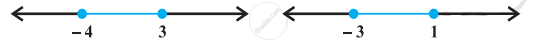• x ∈ (– oo, – 4] ∪ [3, oo)

• x ∈ [– 3, 1]

• x ∈ (– oo, – 4) ∪ [3, oo)

• x ∈ [– 4, 3]

Solved Examples | Q 13 | Page 105

If |x + 3| ≥ 10, then ______.

• x ∈ (– 13, 7]

• x ∈ (– 13, 7]

• x ∈ (– oo, – 13] ∪ [7,  oo)

• x ∈ [–  oo, – 13] ∪ [7,  oo)

#### State whether the following statement is True or False:

Solved Examples | Q 14.(i) | Page 105

If x > y and b < 0, then bx < by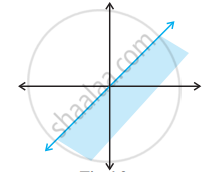• True

• False

Solved Examples | Q 14.(ii) | Page 105

If xy > 0, then x > 0, and y < 0• True

• False

Solved Examples | Q 14.(iii) | Page 105

If xy < 0, then x > 0, and y > 0• True

• False

Solved Examples | Q 14.(iv) | Page 105

If x > 5 and x > 2, then x ∈ (5, oo)• True

• False

Solved Examples | Q 14.(v) | Page 105

If |x| < 5, then x ∈ (– 5, 5)• True

• False

Solved Examples | Q 14.(vi) | Page 105

Graph of x > – 2 is• True

• False

Solved Examples | Q 14.(vii) | Page 105

Solution set of x – y ≤ 0 is• True

• False

#### Fill in the blanks in the following:

Solved Examples | Q 15.(i) | Page 106

If x ≥ – 3, then x + 5 ______ 2

Solved Examples | Q 15.(ii) | Page 106

If – x ≤ – 4, then 2x ______ 8

Solved Examples | Q 15.(iii) | Page 106

If 1/(x - 2) < 0, then x ______ 2

Solved Examples | Q 15.(iv) | Page 106

If a < b and c < 0, then a/c ______ b/c

Solved Examples | Q 15.(v) | Page 106

If |x − 1| ≤ 2, then – 1 ______ x ______ 3

Solved Examples | Q 15.(vi) | Page 106

If |3x – 7| > 2, then x ______ 5/3 or x ______ 3

Solved Examples | Q 15.(vii) | Page 106

If p > 0 and q < 0, then p + q ______ p

Exercise [Pages 107 - 113]

### NCERT solutions for Mathematics Exemplar Class 11 Chapter 6 Linear Inequalities Exercise [Pages 107 - 113]

#### Short Answer and Solve for x, the inequalities 1 to 12

Exercise | Q 1 | Page 107

4/(x + 1) ≤ 3 ≤ 6/(x + 1), (x > 0)

Exercise | Q 2 | Page 107

(|x - 2| - 1)/(|x - 2| - 2) ≤ 0

Exercise | Q 3 | Page 107

1/(|x| - 3) ≤ 1/2

Exercise | Q 4 | Page 107

|x − 1| ≤ 5, |x| ≥ 2

Exercise | Q 5 | Page 107

5 ≤ (2 - 3x)/4 ≤ 9

Exercise | Q 6 | Page 107

4x + 3 ≥ 2x + 17, 3x – 5 < – 2.

Exercise | Q 7 | Page 107

A company manufactures cassettes. Its cost and revenue functions are C(x) = 26,000 + 30x and R(x) = 43x, respectively, where x is the number of cassettes produced and sold in a week. How many cassettes must be sold by the company to realise some profit?

Exercise | Q 8 | Page 107

The water acidity in a pool is considerd normal when the average pH reading of three daily measurements is between 8.2 and 8.5. If the first two pH readings are 8.48 and 8.35, find the range of pH value for the third reading that will result in the acidity level being normal.

Exercise | Q 9 | Page 107

A solution of 9% acid is to be diluted by adding 3% acid solution to it. The resulting mixture is to be more than 5% but less than 7% acid. If there is 460 litres of the 9% solution, how many litres of 3% solution will have to be added?

Exercise | Q 10 | Page 107

A solution is to be kept between 40°C and 45°C. What is the range of temperature in degree fahrenheit, if the conversion formula is F = 9/5 C + 32?

Exercise | Q 11 | Page 107

The longest side of a triangle is twice the shortest side and the third side is 2cm longer than the shortest side. If the perimeter of the triangle is more than 166 cm then find the minimum length of the shortest side.

Exercise | Q 12 | Page 107

In drilling world’s deepest hole it was found that the temperature T in degree celcius, x km below the earth’s surface was given by T = 30 + 25(x – 3), 3 ≤ x ≤ 15. At what depth will the temperature be between 155°C and 205°C?

Exercise | Q 13 | Page 108

Solve the following system of inequalities (2x + 1)/(7x - 1) > 5, (x + 7)/(x - 8) > 2

Exercise | Q 14 | Page 108

Find the linear inequalities for which the shaded region in the given figure is the solution set.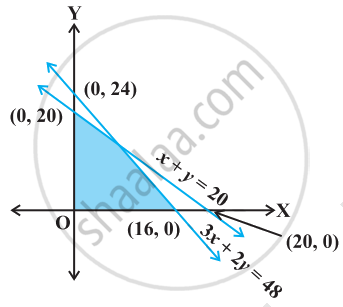Exercise | Q 15 | Page 108

Find the linear inequalities for which the shaded region in the given figure is the solution set.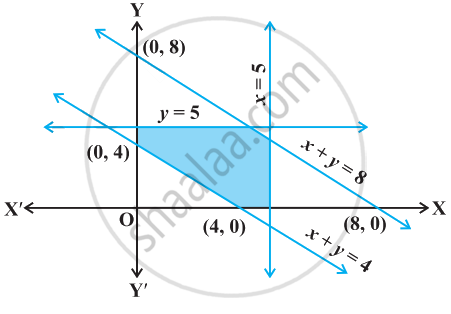Exercise | Q 16 | Page 108

Show that the following system of linear inequalities has no solution x + 2y ≤ 3, 3x + 4y ≥ 12, x ≥ 0, y ≥ 1

Exercise | Q 17 | Page 109

Solve the following system of linear inequalities:

3x + 2y ≥ 24, 3x + y ≤ 15, x ≥ 4

Exercise | Q 18 | Page 109

Show that the solution set of the following system of linear inequalities is an unbounded region 2x + y ≥ 8, x + 2y ≥ 10, x ≥ 0, y ≥ 0

#### Objective Type Questions from 19 to 26

Exercise | Q 19 | Page 109

If x < 5, then ______.

• – x < – 5

• – x ≤ – 5

• – x > – 5

• – x ≥ – 5

Exercise | Q 20 | Page 109

Given that x, y and b are real numbers and x < y, b < 0, then ______.

• x/b < y/b

• x/b ≤ y/b

• x/b > y/b

• x/b ≥ y/b

Exercise | Q 21 | Page 109

If – 3x + 17 < – 13, then ______.

• x ∈ (10, oo)

• x ∈ [10, oo)

• x ∈ (– oo, 10]

• x ∈ [– 10, 10)

Exercise | Q 22 | Page 109

If x is a real number and |x| < 3, then ______.

• x ≥ 3

• – 3 < x < 3

• x ≤ – 3

• – 3 ≤ x ≤ 3

Exercise | Q 23 | Page 109

x and b are real numbers. If b > 0 and |x| > b, then ______.

• x ∈ (– b, oo)

• x ∈ [– oo, b)

• x ∈ (– b, b)

• x ∈ (– oo, – b) ∪ (b, oo)

Exercise | Q 24 | Page 109

If |x −1| > 5, then ______.

• x ∈ (– 4, 6)

• x ∈ [– 4, 6]

• x ∈ [– oo, – 4) ∪ (6, oo)

• x ∈ [– oo, – 4) ∪ [6, oo)

Exercise | Q 25 | Page 109

If |x + 2| ≤ 9, then ______.

• x ∈ (– 7, 11)

• x ∈ [– 11, 7]

• x ∈ (– oo, – 7) ∪ (11, oo)

• x ∈ (– oo, – 7) ∪ [11, oo)

Exercise | Q 26 | Page 110

The inequality representing the following graph is ______.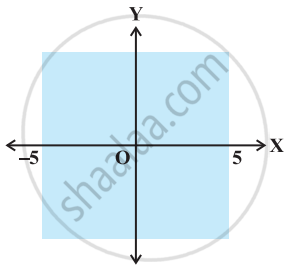• |x| < 5

• |x| ≤ 5

• |x| > 5

• |x| ≥ 5

#### Objective the Questions from 27 to 30

Exercise | Q 27 | Page 110

Solution of a linear inequality in variable x is represented on number line in ______.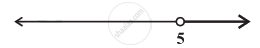• x ∈ (– oo, 5)

• x ∈ (– oo, 5]

• x ∈ [5, oo)

• x ∈ (5, oo)

Exercise | Q 28 | Page 110

Solution of a linear inequality in variable x is represented on number line in ______.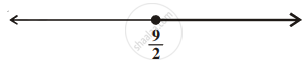• x ∈ (9/2, oo)

• x ∈ [9/2, oo)

• x ∈ [-oo, 9/2)

• x ∈ (-oo, 9/2]

Exercise | Q 29 | Page 110

Solution of a linear inequality in variable x is represented on number line in ______.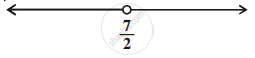• x ∈ (- oo, 7/2)

• x ∈ (-oo, 7/2]

• x ∈ [7/2, -oo)

• x ∈ (7/2, oo)

Exercise | Q 30 | Page 110

Solution of a linear inequality in variable x is represented on number line in ______.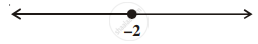• x ∈ (– oo, – 2)

• x ∈ (– oo, – 2]

• x ∈ (– 2, oo]

• x ∈ [– 2, oo)

#### State whether the following statement is True or False:

Exercise | Q 31.(i) | Page 111

If x < y and b < 0, then x/"b" < y/"b"

• True

• False

Exercise | Q 31.(ii) | Page 111

If xy > 0, then x > 0 and y < 0

• True

• False

Exercise | Q 31.(iii) | Page 111

If xy > 0, then x < 0 and y < 0

• True

• False

Exercise | Q 31.(iv) | Page 111

If xy < 0, then x < 0 and y < 0

• True

• False

Exercise | Q 31.(v) | Page 111

If x < –5 and x < –2, then x ∈ (– ∞, – 5)

• True

• False

Exercise | Q 31.(vi) | Page 111

If x < –5 and x > 2, then x ∈ (– 5, 2)

• True

• False

Exercise | Q 31.(vii) | Page 111

If x > –2 and x < 9, then x ∈ (– 2, 9)

• True

• False

Exercise | Q 31.(viii) | Page 111

If |x| > 5, then x ∈ (– oo, – 5) ∪ [5, oo)

• True

• False

Exercise | Q 31.(ix) | Page 111

If |x| ≤ 4, then x ∈ [– 4, 4]

• True

• False

Exercise | Q 31.(x) | Page 111

Graph of x < 3 is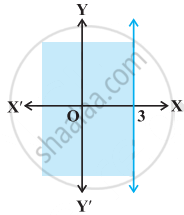• True

• False

Exercise | Q 31.(xi) | Page 111

Graph of x ≥ 0 is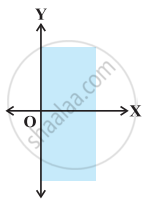• True

• False

Exercise | Q 31.(xii) | Page 112

Graph of y ≤ 0 is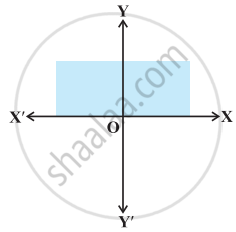• True

• False

Exercise | Q 31.(xiii) | Page 112

Solution set of x ≥ 0 and y ≤ 0 is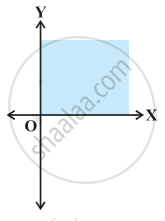• True

• False

Exercise | Q 31.(xiv) | Page 112

Solution set of x ≥ 0 and y ≤ 1 is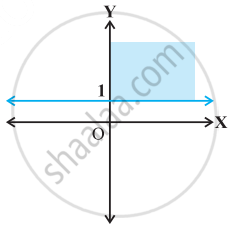• True

• False

Exercise | Q 31.(v) | Page 113

Solution set of x + y ≥ 0 is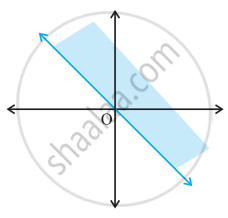• True

• False

#### Fill in the blanks of the following:

Exercise | Q 32.(i) | Page 113

If – 4x ≥ 12, then x ______ – 3.

Exercise | Q 32.(ii) | Page 113

If (-3)/4 x ≤ – 3, then x ______ 4.

Exercise | Q 32.(iii) | Page 113

If 2/(x + 2) > 0, then x  ______ –2.

Exercise | Q 32.(iv) | Page 113

If x > – 5, then 4x ______ –20.

Exercise | Q 32.(v) | Page 113

If x > y and z < 0, then – xz ______ – yz.

Exercise | Q 32.(vi) | Page 113

If p > 0 and q < 0, then p – q ______ p.

Exercise | Q 32.(vii) | Page 113

If |x + 2| > 5, then x ______ – 7 or x ______ 3.

Exercise | Q 32.(viii) | Page 113

If – 2x + 1 ≥ 9, then x ______ – 4.

## Chapter 6: Linear Inequalities

Solved ExamplesExercise## NCERT solutions for Mathematics Exemplar Class 11 chapter 6 - Linear Inequalities

NCERT solutions for Mathematics Exemplar Class 11 chapter 6 (Linear Inequalities) include all questions with solution and detail explanation. This will clear students doubts about any question and improve application skills while preparing for board exams. The detailed, step-by-step solutions will help you understand the concepts better and clear your confusions, if any. Shaalaa.com has the CBSE Mathematics Exemplar Class 11 solutions in a manner that help students grasp basic concepts better and faster.

Further, we at Shaalaa.com provide such solutions so that students can prepare for written exams. NCERT textbook solutions can be a core help for self-study and acts as a perfect self-help guidance for students.

Concepts covered in Mathematics Exemplar Class 11 chapter 6 Linear Inequalities are Inequalities - Introduction, Algebraic Solutions of Linear Inequalities in One Variable and Their Graphical Representation, Graphical Solution of Linear Inequalities in Two Variables, Solution of System of Linear Inequalities in Two Variables.

Using NCERT Class 11 solutions Linear Inequalities exercise by students are an easy way to prepare for the exams, as they involve solutions arranged chapter-wise also page wise. The questions involved in NCERT Solutions are important questions that can be asked in the final exam. Maximum students of CBSE Class 11 prefer NCERT Textbook Solutions to score more in exam.

Get the free view of chapter 6 Linear Inequalities Class 11 extra questions for Mathematics Exemplar Class 11 and can use Shaalaa.com to keep it handy for your exam preparation